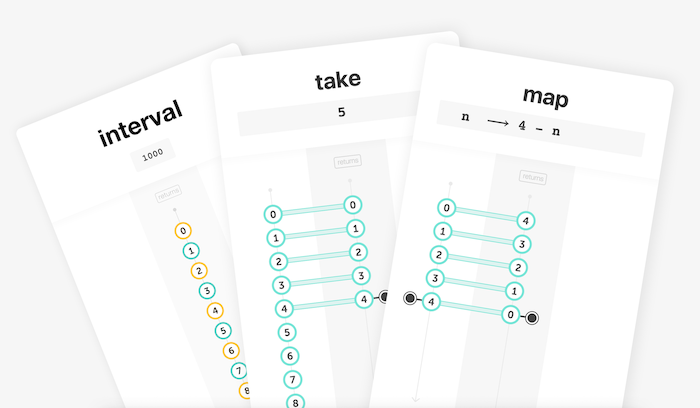Pipeable operators - Build your own with RxJS!

Today, I will use RxJS pipeable operators and create a custom and reusable operator. This is based on the demo I made in Episode 27.

Let’s print this in the console:with those three cards:## Part 1 - Reminder: stream, reactivity and immutability

New to Reactive Programming? Start with Episode 1. Here is a summary.

A stream is a sequence of events over time (eg. a stream of click events). In this episode, I’ll use `❚ interval` to create a stream that emits incremental numbers, periodically.

A listener reacts to events emitted by a stream (values, error and completion notification). This is the reactivity principle. I’ll use `console.log` as a listener to react to the emitted values.

Operators transform, filter and combine streams. An operator never modifies the input stream. Instead, it returns a new stream. This is the immutability principle. To create a “gaussian” stream from interval I need:

• the mapping operator `❚ map`. It projects each event of the input stream with a project function.
• the filtering operator `❚ take` to set a maximum amount of events to emit. Indeed, interval emits an infinite number of events, but I want to print only 25 lines on the console.

## Part 2 - Implementation with RxJS pipeable operators

This example is based on RxJS v6.0 and pipeable operators. Download other versions with my explorer:

Import the creation function `❚ interval` and the pipeable operators `❚ map` and `❚ take`:

``````import { interval } from "rxjs";
import { map, take } from "rxjs/operators";
``````The creation function `❚ interval` returns an `Observable`. It has a built-in `pipe` method to chain pipeable operators.

Emit incremental numbers and complete immediately after the 25th value:

``````const stream1 = interval(350).pipe(
take(25)
);
``````

Emit values projected with a gaussian function, every 350ms:

``````const stream2 = interval(350).pipe(
take(25),
map(gaussian)
);
``````

Emit lines of “•” based on decimals:

``````const stream3 = interval(350).pipe(
take(25),
map(gaussian),
map(num => "•".repeat(Math.floor(num * 65)))
);
``````

Use the `subscribe` method to pass a stream listener:

``````stream.subscribe(console.log)
``````

## Part 3 - Creation of a reusable and custom operator

What if I want both a gaussian stream of `•` and a bezier stream of `~`?

``````const gaussian_stream = interval(350).pipe(
take(25),
map(gaussian),
map(num => "•".repeat(Math.floor(num * 65)))
);

const bezier_stream = interval(350).pipe(
take(25),
map(bezier),
map(num => "~".repeat(Math.floor(num * 65)))
);
``````

Clearly, this code could be refactored. For that, I need to create my own operator, ideally based both on `take(25)` and `map(num => ...)`.

Previously, those two RxJS operators were chained with the pipe method built into `Observable`. But here, I want to chain two operators in a separate function.

To do so, RxJS provides a utility pipe function, that needs to be imported:

``````import { interval, pipe } from "rxjs";
import { map, take } from "rxjs/operators";
``````

I use this pipe function to chain map and take. Et voilà! My custom `draw` operator:

``````const draw = brush =>
pipe(
map(num => brush.repeat(Math.floor(num * 65))),
take(25)
);
``````

Finally, my home-made `draw` operator can be used like any other RxJS pipeable operators:

``````const gaussian_stream = interval(350).pipe(
map(gaussian),
draw("•")
);

const bezier_stream = interval(350).pipe(
map(bezier),
draw("~")
);
``````

## Summary

• Import pipeable operators from `rxjs/operators`
• Chain them with the pipe method built into `Observable`
• Optionally, import the pipe function to build custom and reusable operators from other pipeable operators

You can download the full source code in several versions on reactive.how/rxjs/explorer.